## Register Now

Lorem ipsum dolor sit amet, consectetur adipiscing elit.Morbi adipiscing gravdio, sit amet suscipit risus ultrices eu.Fusce viverra neque at purus laoreet consequa.Vivamus vulputate posuere nisl quis consequat.

Vector Field produced around an electrically charged particle is termed as Electric Field. This post will discuss in detail about what is an Electric Field, what are Electric Field Lines, Gauss’s Law, Coulomb’s Law and their various Applications.

## What is Electric Field

By definition, an Electric Field is an Electric Force per one unit of charge (one coulomb). Since it is a Force, it is a vector and therefore it has both magnitude and direction. Electrical Field strength is used to describe its vectors (magnitude and direction). Electrical energy is enclosed in Electrical Fields.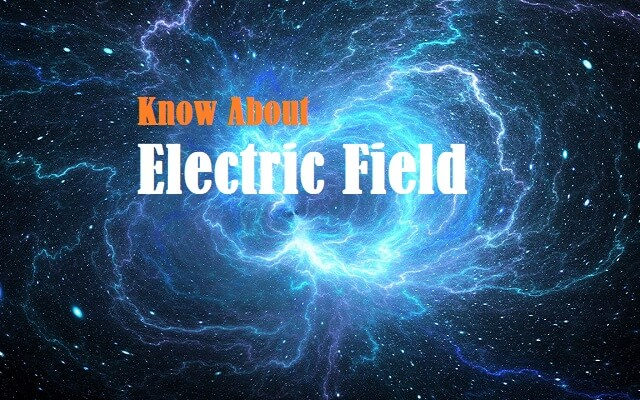Fig. 1 – Introduction to Electric Field

Electrical Field strength can be defined as the point in the Electrical Field being equal to the force that is exerted on a small unit charge placed at that point. The strength of an Electrical Field is also spoken of as its intensity; it is the intensity of the Electrical Field at one particular location.

Let us consider a particle ‘Q’ with a positive charge ‘q’, then the Electrical Field value E can be defined in terms of Force F experienced by a particle with charge q is;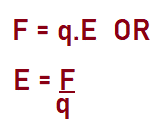Both F and E have magnitude and direction as they are vector quantities. For a positively charged particle, the direction of Electric Field is equal to the direction of Force.

Similarly, for a negatively charged particle, the Force is opposite to the Electrical Field.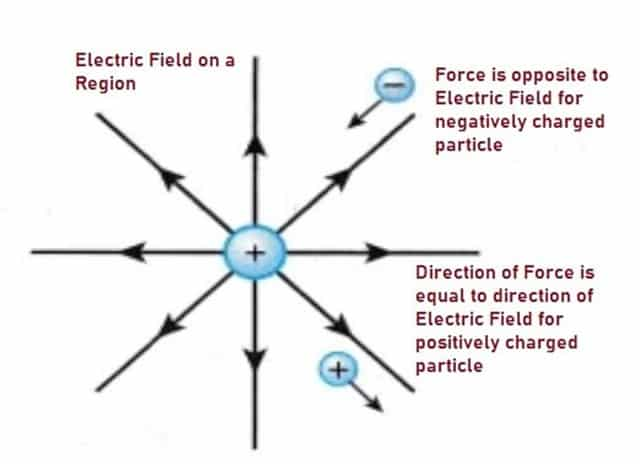Fig. 2 – Representation of Electrical Field and Force Vectors

## Electric Field Lines

Field Lines are a pattern of lines that help in visualizing Electrical Fields and other Vector Fields. This concept was proposed by Michael Faraday who felt that Electrical Fields could be interpreted using Field Lines instead of Vector Diagrams.

They are depicted as starting on positive charges and terminating on negative charges. There are three types of Electrical Field Lines namely:

• Electric Field Lines for Positive Charge
• Electric Field Lines for Negative Charge
• Electric Field Lines for Dipole

### Electric Field Lines for a Positive Charge

As we know, for a positively charged particle, the direction of Electrical Field is equal to the direction of Force and hence the electrical field lines will point away from the positive charge. More field lines mean that the Electrical Field is stronger. The intensity of the electrical field weakens as we move away from the charge.

### Electric Field Lines for a Negative Charge

For a negatively charged particle, the Force is opposite to the Electric Field and hence the Field lines will point inwards.

### Electric Field Lines for Dipole

A Dipole is created when two opposite electrical charges are separated. It is quantitated by a Dipole Moment (μ). The field lines drawn are rotationally symmetrical.

There are two types of Dipoles:

• Electric Dipole
• Magnetic Dipole

#### Electric Dipole

This happens when protons and electrons are separated from each other. They can be separated by a small distance. Separation can be temporary or permanent. In the permanent case, this electric Dipole is called an Electret.

#### Magnetic Dipole

This can be created when an electric current is contained in a closed loop. Electric charges also have their own magnetic fields associated with them. The direction of this Dipole moment follows the right-hand grip rule. It is calculated by multiplying the current of the loop by the area of the loop.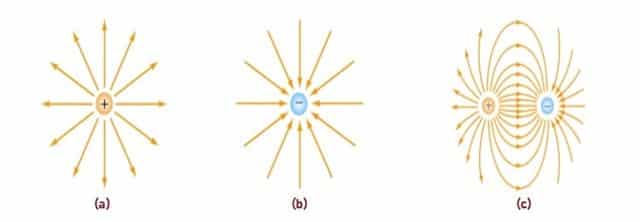Fig. 3 – Electrical Field for a) Positive Charge b) Negative Charge c) Dipole

## Laws of Electric Field

The two laws are:

• Gauss’s Law
• Coulomb’s Law

### Gauss’s Law

This law was stated by German mathematician Carl Friedrich Gauss however it was published posthumously as part of his collection of works. This law describes the static Electric Field that is generated by a distribution of electric charges. It states that:

The electric flux through any closed surface is directly proportional to the total electric charge enclosed by the surface i.e. a positive charge generates a positive Electrical Field. This law is represented in the form of equation:

∫V∇⋅e dv=Sen^ da=Qε0

Where:

e = Electric Field

Q = Enclosed electric charge

ε0 = Electric Permittivity of free space

n^ = Outward pointing unit normal

S = Surface Integral.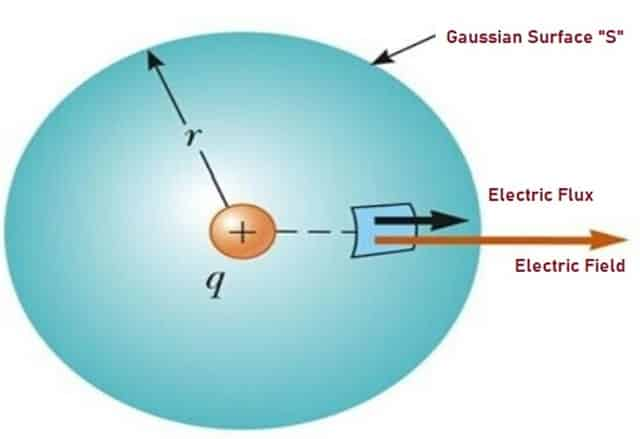Fig. 4 – Representation of Gauss’s Law

### Coulomb’s Law

Coulomb’s Law quantitates the amount of force between two stationary and electrically charged objects. It states that:

The product of the quantity of charge on the objects is directly proportional to the electrical force between them and inversely proportional to the square of the distance of separation between the two objects.

This law is represented in the form of equation:

F= k • q1 • q2 / d2

Where:

F = Electrostatic force between two objects.

k = 2 Coulombs constant.

q = Quantity of charge for object 1 and object 2.

d = Distance between the objects.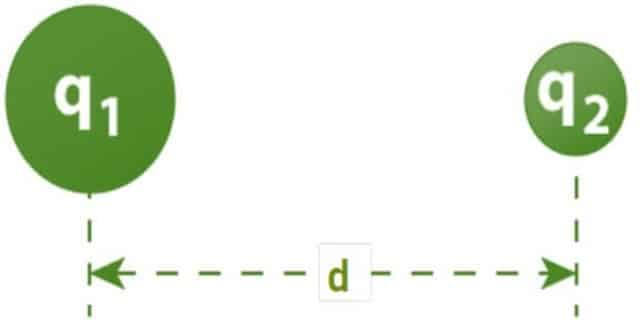Fig. 5 – Representation of Coulomb’s Law

## Applications of Electrical Field

Applications include:

• It is used in Tissue engineering which involves the creation of artificial tissues.
• They are also used in the medical industry for distinguishing the properties of certain types of cancerous and non-cancerous tissues.
• Electrospinning is also another section in medicine that uses Electric Field. Electric-Fields are generated through high voltages between the capillaries and a counter electrode.
• This principle is applied in Aerodynamic Engineering, Pyrolysis and Gasification processes. Process engineering uses Electric-Fields for the reduction of Co, pollutant emissions and environmental contaminants.
• They also shield the earth from cosmic radiation – that sure makes them very useful!
• Cars, airplanes and generators need Electrical Fields to work too since they need batteries, alternators and magneto to work.
```Also Read:
First Aid Treatment in Electric Shock – Causes, Sources, Severity
Electric Car – Types, Components, How it Works and Advantages
Hydroelectric Power Plant – Classification, Working & Applications```